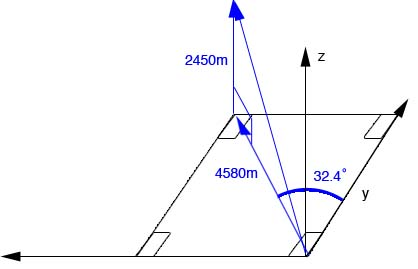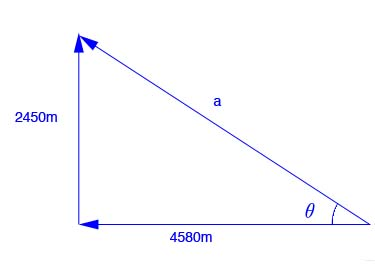SEARCH HOMEMath Central Quandaries & Queriesjenina, a student: The summit of a mountain, 2450m above base camp, is measured on a map to be 4580m horizontally from the camp in a direction 32.4º west of north. What are the components of the displacement vector from camp to summit? What is its magnitude? Choose the x axis east, y axis north and z axis up.Hi Jenina,

Here is an aproximate diagram of your problem:If you look at a 2-dimensional view of the vectors, we can see that you simply can use Pythagoras' theorem to find the magnitude of the displacement vector and trig to find the direction.Remember your direction will include the both compass direction and elevation. Displacement in the x and y direction are a component of the horizontal direction and displacement. Displacement in the z direction is a component of the magnitude of the direction vector.

Hope this helps,

JaniceMath Central is supported by the University of Regina and The Pacific Institute for the Mathematical Sciences.